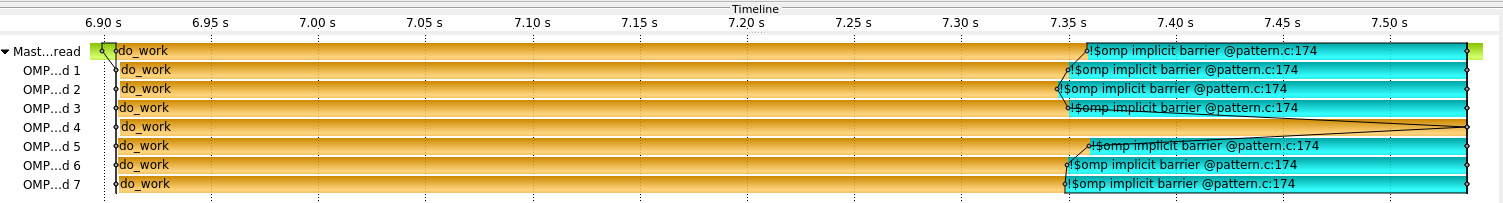# Load imbalance due to computational complexity (unknown a priori)

Usual symptom(s):
• Load Balance Efficiency: The Load Balance Efficiency (LBE) is computed as the ratio between Average Useful Computation Time (across all processes) and the Maximum Useful Computation time (also across all processes). (more...)

In some algorithms it is possible to divide the whole computation into smaller, independent subparts. These subparts may then be executed in parallel by different workers. Even though the data, which is worked on in each subpart, might be well balanced in terms of memory requirements there may be a load imbalance of the workloads. This imbalance may occur if the computational complexity of each subpart depends on the actual data and cannot be estimated prior to execution.

This pattern may appear within an outer iterative structure. In some cases the distribution of work is different in each such iteration. In other cases there may be some locality of computational cost (i.e., the work units with a higher computational complexity may always be the same or change slowly).From a high level perspective the structure of the code can be abstracted as:

#pragma omp parallel for
for (i=0; i<N; i++) {
do_work(i);    // actual amount of work depends on i but not known a priori
}

Recommended best-practice(s):
Related program(s):